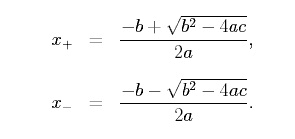# Quadratic Equations Roots using C++ program

Quadratic equations are in the form- a(x*x) + b(x) + c =0. Finding the value of x which satisfies the equation are called roots. Let's find them using C++ program.

Quadratic equations are in the form:- a(x*x) + b(x) + c =0. Finding the value of x which satisfies the equation are called solutions for given quadratic equations or roots of the given equation.

The formula for calculating the roots manually is:-How to use code:-

The code file is attached you can copy the code and paste it into your preferred IDE. Our suggestion will be to use VS Code.